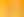Algebra in Action: Patterns, Generalisations and Relationships

# Stages1. Rules, Rules, Rules

EXPLORE

Suggested Learning Intentions

• To use a pattern to find an unknown
• To determine an unknown using pronumerals
• To explore, construct and explain generalisations between two variables

Sample Success Criteria

• I can describe a pattern
• I can determine an unknown using pronumerals
• I can create a rule to describe a relationship between two variables
• I can model and justify my thinking using a range of manipulatives
EXPLORE

2. Garden Beds

EXPLORE

Suggested Learning Intentions

• To use a pattern to find an unknown
• To describe an unknown using pronumerals
• To explore, construct and explain generalisations between two variables
• To investigate, interpret and analyse graphs
• To connect algebraic expressions and their graphical representations
• To understand and justify that there is more than one way to describe a generalisation

Sample Success Criteria

• I can describe a pattern
• I can use a pattern to find an unknown
• I can determine an unknown using pronumerals
• I can construct generalisations between two variables
• I can create a rule to describe a relationship between two variables
• I can describe the elements of graphs
• I can match algebraic expressions with their graphical representation
• I can represent a generalisation in more than one way
• I can use manipulatives to model and justify my solutions
EXPLORE

3. The Snail Problem

EXPLORE

Suggested Learning Intentions

• To use a pattern to find an unknown
• To describe an unknown using pronumerals
• To solve linear and non-linear equations using algebraic and graphical techniques
• To compare and contrast the elements of linear and non-linear equations
• To successfully apply a range of problem-solving strategies to a given task

Sample Success Criteria

• I can describe a pattern
• I can model a pattern using concrete materials
• I can determine an unknown using pronumerals
• I can graph algebraic expressions
• I can identify the elements of a graph
• I can use a range of problem-solving strategies
• I can explain and justify my solutions using a variety of manipulatives
EXPLORE

4. Chocolate Boxes

EXPLORE

Suggested Learning Intentions

• To use a pattern to find an unknown
• To describe an unknown using pronumerals
• To explore, construct and explain generalisations between two variables
• To complete a mathematical investigation involving variables
• To successfully apply a range of problem-solving strategies to a given task

Sample Success Criteria

• I can use a pattern to find an unknown
• I can model a pattern using concrete materials
• I can use pronumerals to describe an unknown
• I can describe a relationship between two variables using an algebraic expression
• I can complete a mathematical investigation involving variables
• I can select an appropriate problem-solving strategy from a range of strategies and successfully apply it to a task
• I can use a range of manipulatives to explain and justify my solutions
EXPLORE